# Rectangular garden

The perimeter of Peter's rectangular garden is 98 meters. The width of the garden is 60% shorter than its length.
Find the dimensions of the rectangular garden in meters.
Find the garden area in square meters.

a =  35 m
b =  14 m
S =  490 m2

### Step-by-step explanation: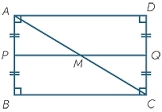Did you find an error or inaccuracy? Feel free to write us. Thank you!Tips to related online calculators
Do you have a linear equation or system of equations and looking for its solution? Or do you have a quadratic equation?
Do you want to convert length units?

#### You need to know the following knowledge to solve this word math problem:

We encourage you to watch this tutorial video on this math problem:

## Related math problems and questions:

• RectangleThe width of the rectangle is 65% of its length. Perimeter of the rectangle is 132 cm. Determine the dimensions of the rectangle.
• PropertyThe length of the rectangle-shaped property is 8 meters less than three times of the width. If we increase the width 5% of a length and lendth reduce by 14% of the width it will increase the property perimeter by 13 meters. How much will the property cost
• RectangleFind the dimensions of the rectangle, whose perimeter is 108 cm and the length is 25% larger than the width.
• Hop-gardenThe length of the rectangular hop garden Mr. Smith increased by 25% and its width by 30%. What is the percentage change in area of hop garden?
• Area of gardenIf the width of the rectangular garden is decreased by 2 meters and its length is increased by 5 meters, the area of the rectangle will be 0.2 ares larger. If the width and the length of the garden will increase by 3 meters, its original size will increas
• PlotThe length of the rectangle is 8 smaller than three times the width. If we increase the width by 5% of the length and the length is reduced by 14% of the width, the circumference of rectangle will be increased by 30 m. What are the dimensions of the recta
• Dimensions of rectangleFind the dimensions of a rectangle given that its perimeter is 110 cm and its length is 1 cm more than twice its width.
• HarryHarry Thomson bought a large land in the shape of a rectangle with a circumference of 90 meters. He divided it into three rectangular plots. The shorter side has all three plots of equal length, their longer sides are three consecutive natural numbers. Fi
• Two gardens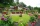The total area of the two gardens is 864 m2. The first garden is 60 m2 smaller than three times the second garden. What is the area of each garden?
• Rectangle areaThe length of a rectangle of x units is increased by 10%, and its width of y units is increased by 15%. What is the ratio of the area of the old rectangle to the area of the new rectangle?
• Garden fence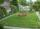The garden has the shape of a rectangular triangle with an area of 96 square meters and a 16 m long one leg. How many meters of the fence need to be fenced?
• Three shapes1/5 of a circle is shaded. The ratio of area if square to the sum of area of rectangle and that of the circle is 1:2. 60% of the square is shaded and 1/3 of the rectangle is shaded. What is the ratio of the area of circle to that of the rectangle?
• Parcel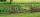Both dimensions of the rectangular parcel were increased by 31%. By how many % has increased its acreage?
• The rectangleDetermine the area of the rectangle where the length and width are in the ratio 5:2 and its length is 7.5 cm longer than its width. Determine also its length and its width.
• A rectangle's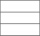A rectangle's length is 3 times more than twice its width. If the perimeter of the rectangle is 60 feet. What is the length of the rectangle
• The circumferenceThe circumference and width of the rectangle are in a ratio of 5: 1. its area is 216cm2. What is its length?
• Square sides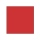If the square side increases by 28%, the square circumference increases by 11.2 meters. Find the length of the original square side.